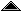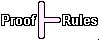# First-Order Predicate Logic

 This is a very simple formal specification based on but not the same as a logic called Q by Hunter in [Hunter71]. The specification is written in Standard ML, a strict functional programming language, and can be considered formal since Standard ML does benefit from a formal semantic specification presented as a structured operational semantics, and is therefore a well defined notation. The specification has been run through the Moscow ML implementation of Standard ML, together with a small example proof. The system is a classical first-order predicate logic with two propositional connectives (not and implies) and a universal quantifier, presented as a "Hilbert style" axiom system in which there are two inference rules, modus ponens and generalisation, and six axiom schemata.

##The only terms are variables. The only atomic formulae are n-ary predicates, for n >= 0. The same name can be used for predicates at several arities. The following is the abstract syntax, of terms and formulae, expressed in Standard ML as a couple of datatypes: ```infix 5 ==>; datatype term = V of string and formula = P of string * term list | Not of formula | op ==> of (formula * formula) | Forall of string * formula; ``` The resulting output from the SML compiler, showing the types of the defined constructors is as follows: ```> infix 5 ==> datatype term datatype formula con V = fn : string -> term con P = fn : string * term General.list -> formula con Not = fn : formula -> formula con ==> = fn : formula * formula -> formula con Forall = fn : string * formula -> formula ```

##We use the Standard ML List type constructor in the following specification to represent sets. In execution this limits these sets to be finite, and also would give incorrect identity criteria for the sets. For the purpose of reading the Standard ML as a specification the finiteness limitation is to be disregarded. Equality over lists will not be used in the specification. The infix function mem is defined to correspond to the membership predicate: ```infix 5 mem; fun (e mem l) = exists (fn x => x = e) l; ``` ```> infix 5 mem > val mem = fn : ''a * ''a General.list -> bool ``` An interpretation is represented by an ML structure with two components. The component named dom is a set of elements which represents the domain of the interpretation. The second component named map assigns to each predicate letter a set of tuples of elements from the domain of interpretation for which the predicate is true under the interpretation. This provides the interpretation of that predicate letter at all arities. It is represented as a list of lists of elements, each of the constituent lists representing a single instance at which the predicate is true. The logic is boolean and therefore the predicate is to be considered false at all other points. ```type 'e interp = {dom:'e list, map:string->'e list list}; ``` To interpret the free variables in a term or formula we need an assignment of elements to the variable names. The semantics of terms simply shows that the meaning of a variable is determined by this assignment (there being in this simplified logic no other kind of term). A function is also defined to evaluate a sequence of terms by evaluating each term in turn, returning a sequence of elements from the domain of interpretation. ```fun teval v (V s) = v s; fun tseval v ts = map (teval v) ts; ``` ```> val teval = fn : (string -> 'a) -> term -> 'a > val tseval = fn : (string -> 'a) -> term General.list -> 'a General.list ``` The truth value of a formula f under an interpretation i is feval i f, where feval is a (higher order) function taking two successive arguments, an interpretation i and a propositional formula f. feval is defined as follows: ```fun fpeval {dom=d, map=m} v (P (p,ts)) = (tseval v ts) mem (m p) | fpeval i v (Not f) = not (fpeval i v f) | fpeval i v (f1 ==> f2) = if (fpeval i v f1) then (fpeval i v f2) else false | fpeval {dom=d, map=m} v (Forall (s, f)) = all (fn e => fpeval {dom=d, map=m} (fn vs => if vs=s then e else v vs) f ) d; ``` The type of fpeval is as shown below taking an interpretation (over ''a, a type variable), a valuation function assigning of elements from the same type to variable names, and a formula, and returning the truth value of the formula in the context of the interpretation and valuation. ```fpeval: (''a interp) -> (string -> ''a) -> formula -> bool; ```

 A formula is tautological (and hence true) if it is true under every interpretation and contradictory (and hence false) if it is false under every interpretation.

## AuxiliaryFunctions

 To express the inference rules for our logic we need to have available specifications of the concept of free variable and for the process of substitution of a term for a variable in a formula. Free Variables ```fun tvar (V t) = t; val tvars = map tvar; fun free (P (s,tl)) = tvars tl | free (Not f) = free f | free (f1 ==> f2) = (free f1) @ (free f2) | free (Forall (s,f)) = filter (fn x => not (x=s)) (free f); ``` ```> val tvar = fn : term -> string > val tvars = fn : term General.list -> string General.list > val free = fn : formula -> string General.list ``` Substitution The substitution process we define must avoid capturing any of the free variables (actually, in this logic there can only be one) in the scope of a bound variable which happens to have the same name. This is done by renaming any bound variable which has the same name as a variable being substituted into a formula, for which purpose the function newvar is introduced, which choses a new name for the bound variable to be renamed. ```fun newvar (vh::vl) = vh ^ (newvar vl) | newvar nil = "'"; fun tsubs t1 (V v) (V t2) = if t2=v then t1 else (V t2); fun subs t (V v) (P (s,tl)) = P (s, map (tsubs t (V v)) tl) | subs t (V v) (Not f) = Not (subs t (V v) f) | subs t (V v) (f1 ==> f2) = (subs t (V v) f1) ==> (subs t (V v) f2) | subs (V t) (V v1) (Forall (v2, f)) = if v1=v2 then (Forall (v2, f)) else if t=v2 then let val nv = newvar (free f) in (Forall (nv, subs (V t) (V v1) (subs (V nv) (V v2) f) ) ) end else (Forall (v2, subs (V t) (V v1) f)); ``` ```> val newvar = fn : string General.list -> string > val tsubs = fn : term -> term -> term -> term > val subs = fn : term -> term -> formula -> formula ```

##The proof system is specified as an abstract type qthm, which amounts to a simple LCF-like implementation of the logic. There is just one inference rule, modus ponens, and three axiom schemata, as follows. ```abstype qthm = |- of formula with ``` Modus Ponens ```fun MP (|- A) (|- (B ==> C)) = if A = B then |- C else |- A | MP t1 t2 = t1; ``` Generalisation ```fun GEN (|- A) v = |- (Forall (v, A)); ``` Propositional Axiom Schemata The three propositional axiom schemata are as follows: ```fun PS1 A B = |- (A ==> (B ==> A)); fun PS2 A B C = |- ((A ==>(B ==> C)) ==> ((A ==> B) ==>(A ==> C))); fun PS3 A B = |- ((not A ==> not B) ==> (B ==> A)); ``` Quantification Axiom Schemata The following three axiom schemata concern quantification theory. There are actually four in Hunter, the fourth serving instead of the generalisation rule, which Hunter omits for metatheoretic reasons. ```fun PS4 A (V x) t = |- (Forall (x, A) ==> (subs t (V x) A)); fun PS5 A s = if s mem free A then PS1 A A else |- (A ==> (Forall (s, A))); fun PS6 A B s = |- (Forall (s, A ==> B) ==> ((Forall (s, A)) ==> (Forall (s, B)))); ``` We also need a special function to extract the formula from a theorem, which completes the specification of the abstract type qthm: ```fun f (|- A) = A; end; ``` The resulting signature for the abstract data type qthm is as follows. Note that the functions we have called inference rules take one or more theorems as parameters, while those we have described as axiom schemata, though taking parameters, including formulae, do not require any theorems. ```> abstype qthm val MP = fn : qthm -> qthm -> qthm val GEN = fn : qthm -> string -> qthm val PS1 = fn : formula -> formula -> qthm val PS2 = fn : formula -> formula -> formula -> qthm val PS3 = fn : formula -> formula -> qthm val PS4 = fn : formula -> term -> term -> qthm val PS5 = fn : formula -> string -> qthm val PS6 = fn : formula -> formula -> string -> qthm val f = fn : qthm -> formula ```

## A Small Proof

 This is an LCF-like ML version of the proof given by Hunter of p ==> p. ```val pp = P ("p",[]); val f1 = pp ==> pp; (* this is the goal *) val L1 = PS1 pp (pp ==> pp); (* |- (pp ==> ((pp ==> pp) ==> pp)) *) val L2 = PS2 pp (pp ==> pp) pp;(* |- (pp ==> ((pp ==> pp) ==> pp)) ==> ((pp ==> (pp ==> pp)) ==> (pp ==> pp)) *) val L3 = MP L1 L2; (* |- ((pp ==> (pp ==> pp)) ==> (pp ==> pp)) *) val L4 = PS1 pp pp; (* |- (pp ==> (pp ==> pp) *) val L5 = MP L4 L3; (* |- (pp ==> pp) *) f1 = f L5; (* this checks that the result is the same as our goal *) ``` ```> val pp = P("p", []) : formula > val f1 = ==>(P("p", []), P("p", [])) : formula > val L1 = : pthm > val L2 = : pthm > val L3 = : pthm > val L4 = : pthm > val L5 = : pthm > val it = true : bool ```©created 1997/10/25 modified 1997/10/26